International
Tables for
Crystallography
Volume D
Physical properties of crystals
Edited by A. Authier

International Tables for Crystallography (2006). Vol. D, ch. 1.1, p. 7

## Section 1.1.3.2. Behaviour under a change of basis

A. Authiera*

aInstitut de Minéralogie et de la Physique des Milieux Condensés, Bâtiment 7, 140 rue de Lourmel, 75015 Paris, France
Correspondence e-mail: aauthier@wanadoo.fr

#### 1.1.3.2. Behaviour under a change of basis

| top | pdf |

A multilinear form is, by definition, invariant under a change of basis. Let us consider, for example, the trilinear form (1.1.3.1). If we change the system of coordinates, the components of vectors x, y, z become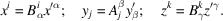Let us put these expressions into the trilinear form (1.1.3.1):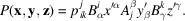Now we can equally well make the components of the tensor appear in the new basis: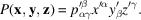As the decomposition is unique, one obtains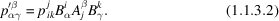One thus deduces the rule for transforming the components of a tensor q times covariant and r times contravariant: they transform like the product of q covariant components and r contravariant components.

This transformation rule can be taken inversely as the definition of the components of a tensor of rank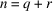.

Example. The operator O representing a symmetry operation has the character of a tensor. In fact, under a change of basis, O transforms into O′: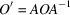so that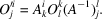Now the matrices A and B are inverses of one another: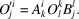The symmetry operator is a tensor of rank 2, once covariant and once contravariant.